International
Tables for
Crystallography
Volume B
Reciprocal space
Edited by U. Shmueli

International Tables for Crystallography (2010). Vol. B, ch. 1.3, pp. 31-32   | 1 | 2 |

## Section 1.3.2.3.9.1. Differentiation

G. Bricognea

aGlobal Phasing Ltd, Sheraton House, Suites 14–16, Castle Park, Cambridge CB3 0AX, England, and LURE, Bâtiment 209D, Université Paris-Sud, 91405 Orsay, France

#### 1.3.2.3.9.1. Differentiation

| top | pdf |

• (a) Definition and elementary properties

If T is a distribution on, its partial derivativewith respect tois defined byfor all. This does define a distribution, because the partial differentiations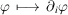are continuous for the topology of.

Suppose thatwith f a locally integrable function such that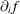exists and is almost everywhere continuous. Then integration by parts along theaxis gives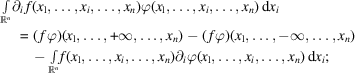the integrated term vanishes, since ϕ has compact support, showing that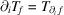.

The test functionsare infinitely differentiable. Therefore, transpositions like that used to definemay be repeated, so that any distribution is infinitely differentiable. For instance,where Δ is the Laplacian operator. The derivatives of Dirac's δ distribution areIt is remarkable that differentiation is a continuous operation for the topology on: if a sequenceof distributions converges to distribution T, then the sequenceof derivatives converges tofor any multi-index p, since asAn analogous statement holds for series: any convergent series of distributions may be differentiated termwise to all orders. This illustrates how robust' the constructs of distribution theory are in comparison with those of ordinary function theory, where similar statements are notoriously untrue.

• (b) Differentiation under the duality bracket

Limiting processes and differentiation may also be carried out under the duality bracketas under the integral sign with ordinary functions. Let the functiondepend on a parameterand a vectorin such a way that all functions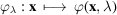be infor all. Letbe a distribution, letand letbe given parameter value. Suppose that, as λ runs through a small enough neighbourhood of,

 (i) all thehave their supports in a fixed compact subset K of; (ii) all the derivativeshave a partial derivative with respect to λ which is continuous with respect to x and λ.

Under these hypotheses,is differentiable (in the usual sense) with respect to λ near, and its derivative may be obtained by differentiation under thesign':• (c) Effect of discontinuities

When a function f or its derivatives are no longer continuous, the derivativesof the associated distributionmay no longer coincide with the distributions associated to the functions.

In dimension 1, the simplest example is Heaviside's unit step function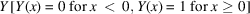: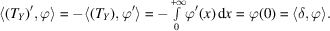Hence, a result long used heuristically' by electrical engineers [see also Dirac (1958)].

Let f be infinitely differentiable for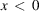and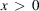but have discontinuous derivativesat[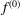being f itself] with jumps. Consider the functions:Theare continuous, their derivativesare continuous almost everywhere [which implies thatandalmost everywhere]. This yields immediately:Thus the distributional derivatives'differ from the usual functional derivativesby singular terms associated with discontinuities.

In dimension n, let f be infinitely differentiable everywhere except on a smooth hypersurface S, across which its partial derivatives show discontinuities. Letanddenote the discontinuities of f and its normal derivativeacross S (bothandare functions of position on S), and letandbe defined by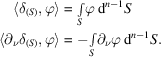Integration by parts shows that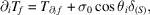whereis the angle between theaxis and the normal to S along which the jumpoccurs, and that the Laplacian ofis given byThe latter result is a statement of Green's theorem in terms of distributions. It will be used in Section 1.3.4.4.3.5to calculate the Fourier transform of the indicator function of a molecular envelope.

### References

Dirac, P. A. M. (1958). The Principles of Quantum Mechanics, 4th ed. Oxford: Clarendon Press.